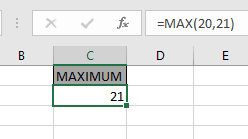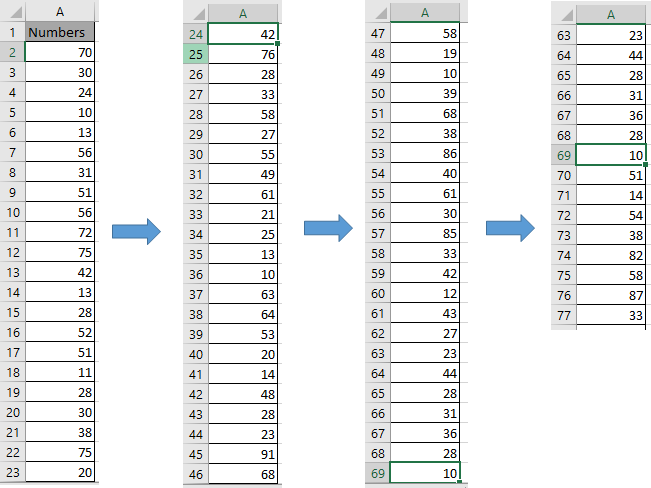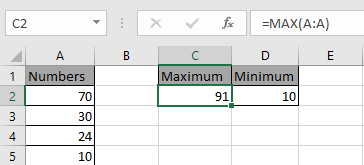# How to use the MAX function in Excel

In this article, we will learn how MAX function works in Excel.
MAX function returns the maximum number is the range.
Syntax:

=MAX(number 1, number 2, ..)

MAX function only considers the numbers
Let’s understand how to add cells in excel 2016 with the example explained here.
Maximum of two input numbers is done via Formula shown below.
Use the formula

=MAX(20,21)Now we wish to find the Maximum from the entire column.
Here we have 76 numbers in column A.Here we need to Maximum value present in this column. There would two methods
Use the formula

=MAX(A2:A77)
OR
=MAX(A:A)As you can see you can get the maximum value from the range of numbers. You can get the minimum value from the range using the MIN function in Excel.

Hope you understood how to get the MAX value using the MAX function in Excel. Explore more articles on Excel number function here. Please state your query in the comment box below.

Related Articles:

How to use MIN function in Microsoft Excel

How to use the LARGE Function in Excel

Excel SMALL function

How To Calculate MODE

How To Calculate Mean

Popular Articles:

50 Excel Shortcuts to Increase Your Productivity

How to use the VLOOKUP Function in Excel

How to use the COUNTIF function in Excel 2016

How to Use SUMIF Function in Excel

Terms and Conditions of use

The applications/code on this site are distributed as is and without warranties or liability. In no event shall the owner of the copyrights, or the authors of the applications/code be liable for any loss of profit, any problems or any damage resulting from the use or evaluation of the applications/code.